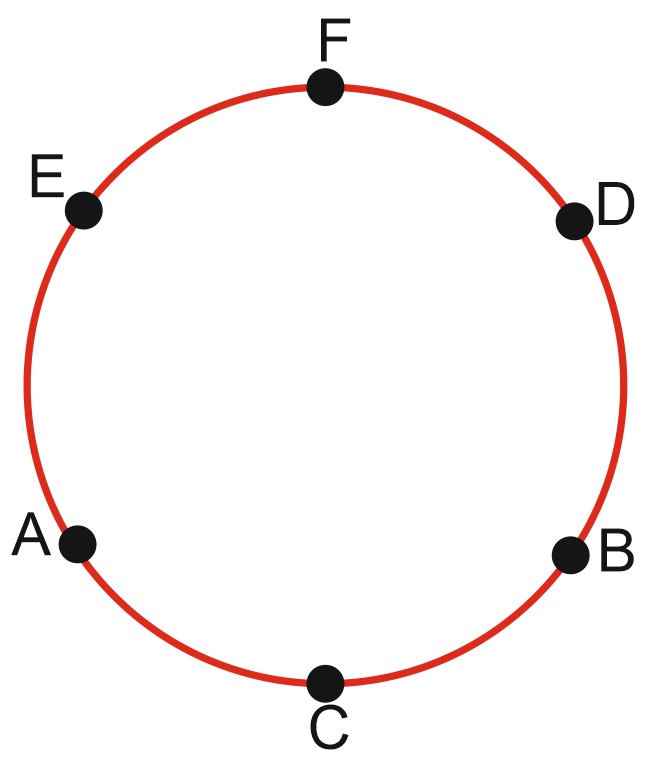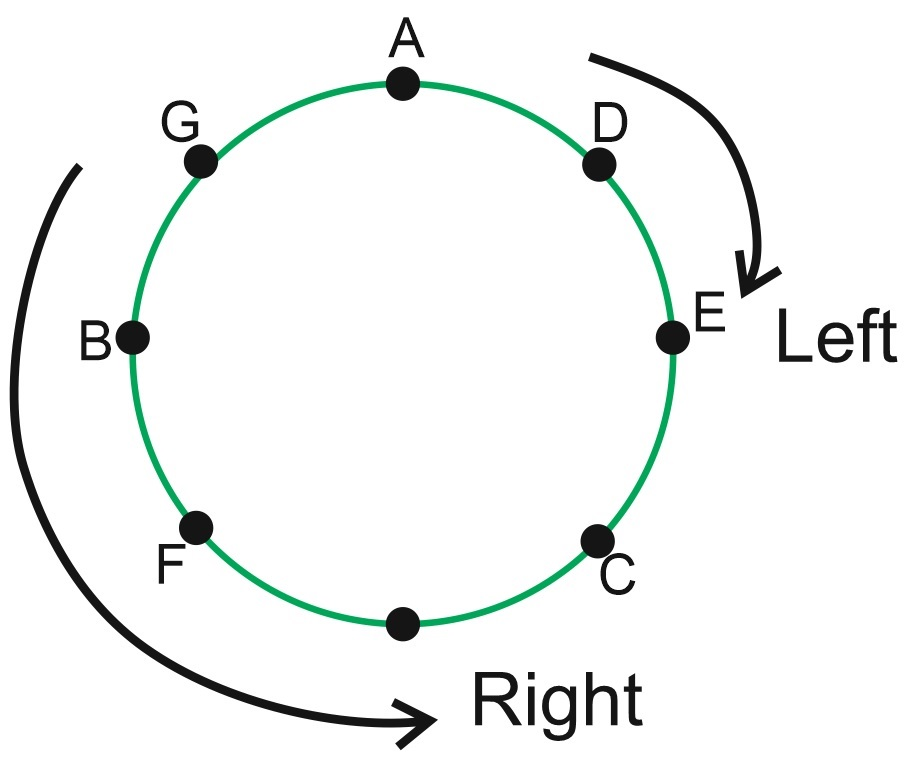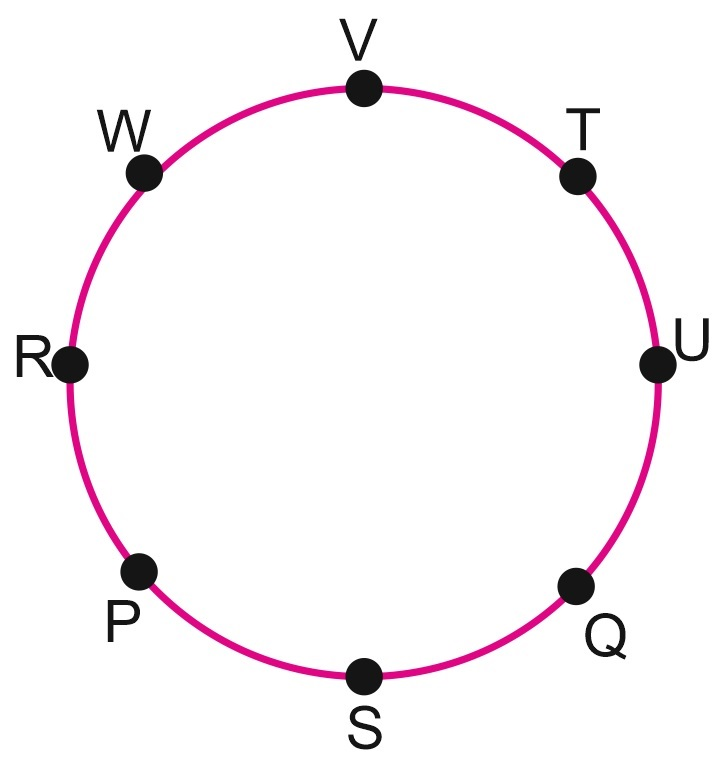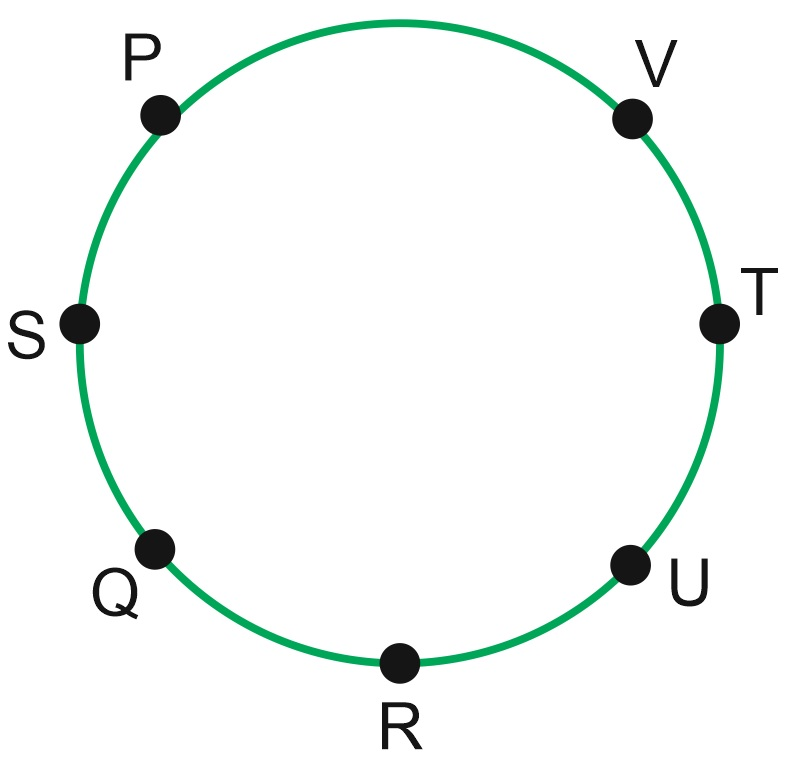Seating Arrangement Questions PDF Download: - Seating Arrangement Plays a vital role in Reasoning Section. In every exam you will get at least 4-5 questions...

sumit Follow

12th March,2020

## Seating Arrangement Problems with Solutions for Competitive Exams

Q.1. Six persons A, B, C, D, E & F are standing in a circle. B is between D & C. A is between E & C. F is at the right of D. Who is between A & F?

(A) E

(B) C

(C) D

(D) None of these.

Ans (A)

SolutionE will be between A & F.

Q.2. A, B, C, D, E, F & G are sitting in a circular region & playing cards. F is 2nd to the right of G. B is neighbour of F but not of C. E is a neighbour of C & is siting 4th at the right of G. D is between E & A.

(1) Who is fourth at left of G ?

(A) D

(B) E

(C) C

(D) B

(2) Who is at left of G ?

(A) A

(B) C

(C) B

(D) F

(3) Who is the neighbour of F ?

(A) E and C

(B) F and B

(C) A and B

(D) C and B

Ans: (1) C, (2) A, (3)D

Solution

When the arrangement is given in circle, then there is a chance of mistake regarding left & right. So, we have to consider our self at the point of starting. Then think about your left & right. So, there will be no mistake.

If we start to solve the problem from left towards the downward, that will be more easier.Note – In this type of question, the word which or who is used for the II person & the words like but, while, whereas, and etc. are used for the I person.

Q.3. Eight friends – P, Q, R, S, T, U, V & W are sitting around a circle facing the center. V is third to the right of Q & second to the left of R. Q is second to the left of T & immediate right of S. U is between Q & T. P is not at the left of R.

(1) Who is exactly at left of S ?

(A) Q

(B) P

(C) U

(D) V

(2) Who is second to right of T ?

(A) R

(B) P

(C) W

(D) V

(3) In which of the following pairs first person sits exactly at right of second persons?

(A) UT

(B) WR

(C) WT

(D) VT

(4) What is the exact position of W?

(A) Just left of V

(B) Just right of V

(C) In between U and V

(D) Immediate right to R

(5) Who is second at right of S?

(A) U

(B) R

(C) T

(D) P

Ans : (1) B, (2)C , (3) D ,(4) B, (5) A

SolutionQ.4. (A) P, Q,R,S,T,U & V are sitting around a circle facing the center.

(B) P is between V and S.

(C) R, who is second to right of S, is between Q and U.

(D) Q is no the neighbour of T.

(1) Which of the following statement is true?

(A) V is between P and S.

(B) S is second to the left of V

(C) R is third to the left of P

(D) P is at immediate left of S

(2) What is the position of T?

(A) between R and V

(B) just left of V

(C) second to the left of R.

(D)second to the right of P

(3) Who is between R and U?

(A) none

(B) S

(C) V

(D) Q

(4) Which of the following statement is wrong?

(A) R is at immediate right of U.

(B) Q is at immediate left of R.

(C) T is third to the right of Q.

(D) U is third to the right of T.

(5) In which of the following pairs, the second member is at the immediate right of the first member?

(A) QS

(B) PV

(C) RU

(D) VT

Ans : (1) D, (2) B, (3) A, (4) A, (5) C

SolutionDirections for Questions 1 to 5: Read the following information carefully and answer the questions given below :

i.            P, Q, R, S, T, U and V are sitting around a circular table facing the centre

ii.            R is next to the left of U and V is second to the left of R.

iii.            P is sitting third to the left of T.

iv.            Q is between S and T.

Q.1. Which of the following is false?

1. P is fourth to the right of T.

2. U is to the immediate right of R.

3. U is third to the right of S.

4. Q is to the immediate left of S.

Q.2. Which of the following is true?

1. R is fourth to the right of T.

2. P is to the immediate right of V.

3. S is second to the left of T.

4. Q is second to the right of V.

Q.3. Which of the following pairs has the first person sitting to the immediate left of the second person?

1. QT

2. RP

3. VS

4. SV

Q.4. In which of the following options, the middle person is sitting between the other two?

1. URT

2. TUQ

3. STQ

4. None of these

Q.5. What is the position of U?

1. Fourth to the right of S

2. To the immediate left of R

3. Between P and T

4. To the immediate right of P

Directions for Questions 6 to 10: Read the following information carefully and answer these questions:

i.            K, L, M, N, O, P and Q are sitting in a circle facing at the centre and playing cards.

ii.            O is neighbour of K and N.

iii.            Q is not between P and M.

iv.            P is to the immediate right of K.

v.            L is second to the left of Q.

bb

1. LK

2. ML

3. NO

4. QN

Q.7. Which of the following pairs has the second person sitting immediately to the right of the first?

1. KL

2. ML

3. OK

4. None of these

Q8. What is the position of P?

1. Second to the left of M

2. Second to the right of M

3. To the immediate left of K

4. To the immediate right of L

Q9. Who are the neighbours of L?

1. K and P

2. M and N

3. P and M

4. None of these

Q. 10 Which of the following persons are sitting adjacent to each other in clockwise order as shown?

1. LQM

2. PLM

3. MNQ

4. ONQ

You can download the PDF files of seating arrangment questions for Banking exams from the link given below:-

>
share